# 15.) a.)In the laboratory, a general chemistry student measured the pH of a 0.376 M aqueous...

15.) a.)In the laboratory, a general chemistry student measured the pH of a 0.376 M aqueous solution of nitrous acid to be 1.872.

Use the information she obtained to determine the Ka for this acid.

Ka(experiment) =

b.)In the laboratory, a general chemistry student measured the pH of a 0.376 M aqueous solution of nitrous acid to be 1.870.

Use the information she obtained to determine the Ka for this acid.

Ka(experiment) =

c.)In the laboratory, a general chemistry student measured the pH of a 0.376 M aqueous solution of hydrocyanic acid to be 4.928.

Use the information she obtained to determine the Ka for this acid.

Ka(experiment) =

15)

a) [HNO2] = 0.376 M

pH = -log[H+] = 1.872

[H+] = 0.01343 M

[H+] = [A-] = 0.01343 M

[HNO2] at equilibrium = 0.376 - 0.01343 = 0.3626 M

Ka (experimental) = [H+][A-]/[HA]

= (0.01343)^2/(0.3626)

= 4.97 x 10^-4

---

b) [HNO2] = 0.376 M

pH = -log[H+] = 1.870

[H+] = 0.01350 M

[H+] = [A-] = 0.01350 M

[HNO2] at equilibrium = 0.376 - 0.01350 = 0.3625 M

Ka (experimental) = [H+][A-]/[HA]

= (0.01350)^2/(0.3625)

= 5.03 x 10^-4

--

c) [HCN] = 0.376 M

pH = -log[H+] = 4.928

[H+] = 1.18 x 10^-5 M

[H+] = [A-] = 1.18 x 10^-5 M

[HCN] at equilibrium = 0.376

Ka (experimental) = [H+][A-]/[HA]

= (1.18 x 10^-5)^2/(0.376)

= 3.70 x 10^-10

#### Earn Coin

Coins can be redeemed for fabulous gifts.

Similar Homework Help Questions
• ### In the laboratory, a general chemistry student measured the pH of a 0.488 M aqueous solution...

In the laboratory, a general chemistry student measured the pH of a 0.488 M aqueous solution of hydrofluoric acid to be 1.711. Use the information she obtained to determine the Ka for this acid. Ka(experiment) = In the laboratory, a general chemistry student measured the pH of a 0.488 M aqueous solution of benzoic acid, C6H5COOH to be 2.273. Use the information she obtained to determine the Ka for this acid. Ka(experiment) = In the laboratory, a general chemistry student...

• ### In the laboratory, a general chemistry student measured the pH of a 0.506 M aqueous solution...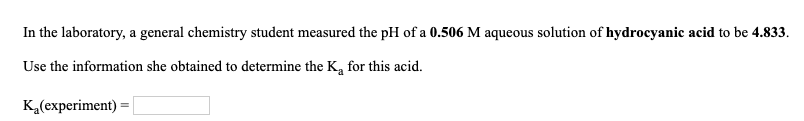In the laboratory, a general chemistry student measured the pH of a 0.506 M aqueous solution of hydrocyanic acid to be 4.833. Use the information she obtained to determine the K, for this acid. K (experiment) In the laboratory, a general chemistry student measured the pH of a 0.540 M aqueous solution of benzoic acid, CH3COOH to be 2.218. Use the information she obtained to determine the K, for this acid. K (experiment)

• ### In the laboratory, a general chemistry student measured the pH of a 0.579 M aqueous solution...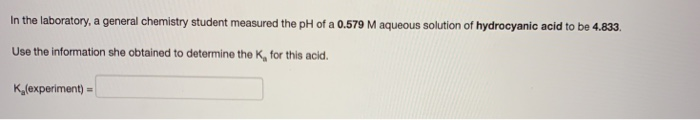In the laboratory, a general chemistry student measured the pH of a 0.579 M aqueous solution of hydrocyanic acid to be 4.833. Use the information she obtained to determine the K, for this acid. K(experiment)

• ### In the laboratory, a general chemistry student measured the pH of a 0.430 M aqueous solution...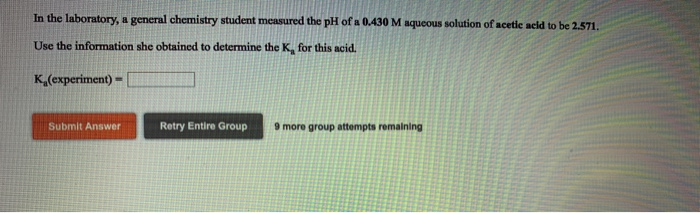In the laboratory, a general chemistry student measured the pH of a 0.430 M aqueous solution of acetie acid to be 2.571. Use the information she obtained to determine the K, for this acid. Ka(experiment)- Submit Answer Retry Entire Group 9 more group attempts remaining In the laboratory a student measures the percent ionization of a 0.430 M solution of acetic acid to be 0626 %. Calculate value of Ka from this experimental data. Ka-l In the laboratory, a general...

• ### In the laboratory, a general chemistry student measured the pH of a 0.392 M aqueous solution...

In the laboratory, a general chemistry student measured the pH of a 0.392 M aqueous solution of formic acid, HCOOH to be 2.059.   Use the information she obtained to determine the Ka for this acid.   Ka(experiment) =

• ### In the laboratory, a general chemistry student measured the pH of a 0.464 M aqueous solution...

In the laboratory, a general chemistry student measured the pH of a 0.464 M aqueous solution of formic acid, HCOOH to be 2.057.   Use the information she obtained to determine the Ka for this acid.   Ka(experiment) =

• ### 1. a. In the laboratory, a general chemistry student measured the pH of a 0.331 M aqueous solution of hypochlorous acid...

1. a. In the laboratory, a general chemistry student measured the pH of a 0.331 M aqueous solution of hypochlorous acid to be 3.986. Use the information she obtained to determine the Ka for this acid. b.In the laboratory, a general chemistry student measured the pH of a 0.331 M aqueous solution of acetylsalicylic acid (aspirin), HC9H7O4 to be 1.985. Use the information she obtained to determine the Ka for this acid.

• ### Please do I do rate :) In the laboratory, a general chemistry student measured the pH...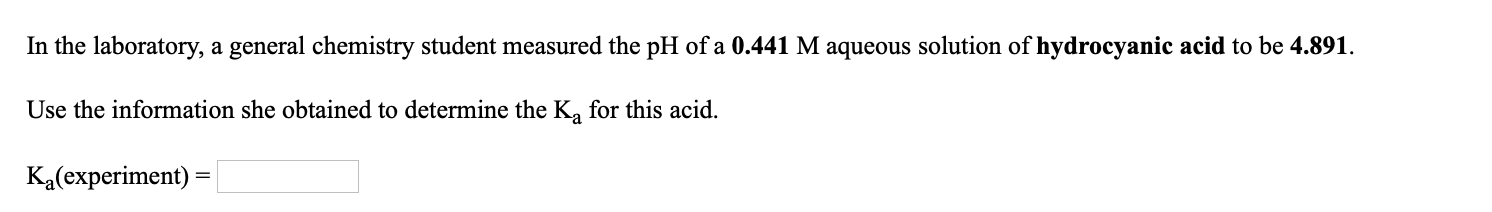Please do I do rate :) In the laboratory, a general chemistry student measured the pH of a 0.441 M aqueous solution of hydrocyanic acid to be 4.891. Use the information she obtained to determine the Ką for this acid. Ka(experiment) =

• ### In the laboratory, a general chemistry student measured the pH of a 0.507 M aqueous solution...

In the laboratory, a general chemistry student measured the pH of a 0.507 M aqueous solution of acetic acid to be 2.504.   Use the information she obtained to determine the Ka for this acid.

• ### In the laboratory, a general chemistry student measured the pH of a 0.370 M aqueous solution...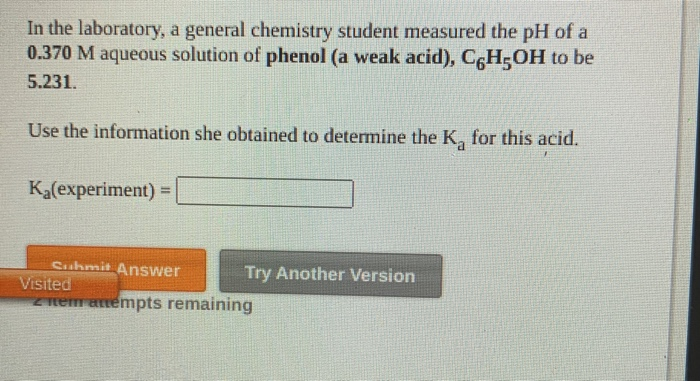In the laboratory, a general chemistry student measured the pH of a 0.370 M aqueous solution of phenol (a weak acid), C6H OH to be 5.231. Use the information she obtained to determine the K, for this acid. Ka(experiment) = Cushmit Answer Try Another Version Visited tremenempts remaining